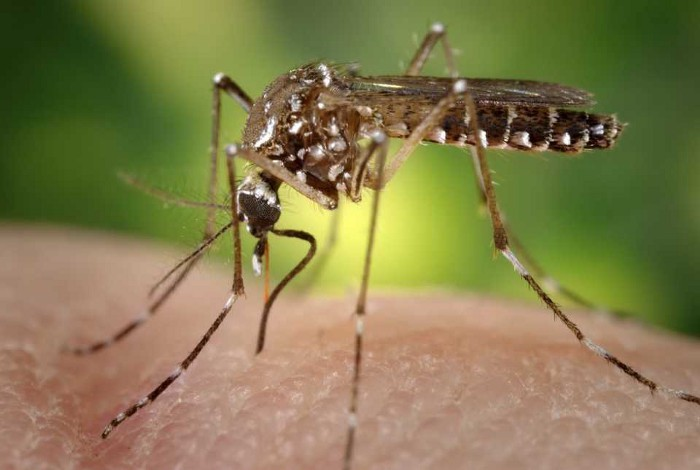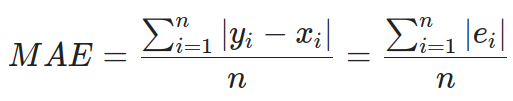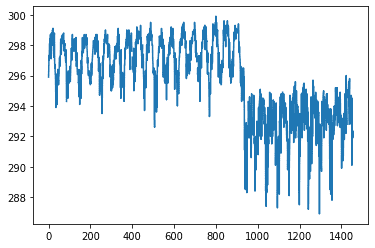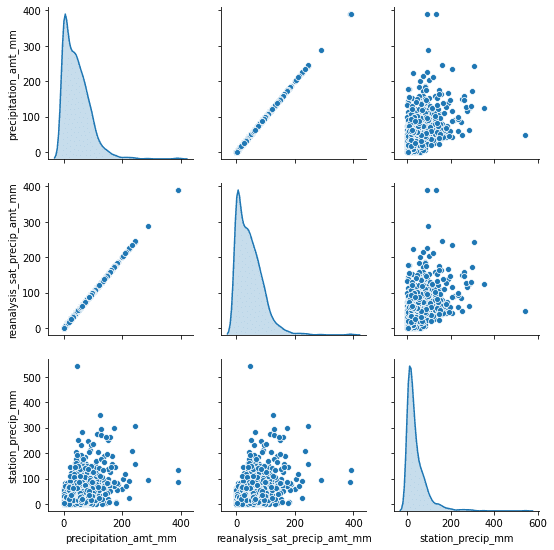# 如何进行数据科学竞赛？（EDA）### 数据集

• city ：sj代表San Juan（圣胡安），iq代表Iquitos
• week_start_date -以yyyy-mm-dd格式给出的日期

NOAA的GHCN每日气候数据气象站测量：

• station_max_temp_c-最高温度
• station_min_temp_c-最低温度
• station_avg_temp_c-平均温度
• station_precip_mm-总降水量
• station_diur_temp_rng_c-昼间温度范围

• precipitation_amt_mm-总降水量

NOAA的NCEP气候预报系统分析测量（0.5×0.5度的标度）

• reanalysis_sat_precip_amt_mm-总降水量
• reanalysis_dew_point_temp_k-平均露点温度
• reanalysis_air_temp_k-平均气温
• reanalysis_relative_humidity_percent-平均相对湿度
• reanalysis_specific_humidity_g_per_kg-平均特定湿度
• reanalysis_precip_amt_kg_per_m2-总降水量
• reanalysis_max_air_temp_k=最大空气温度
• reanalysis_min_air_temp_k-最低空气温度
• reanalysis_avg_temp_k-平均气温
• reanalysis_tdtr_k-白天温度范围

NOAA的CDR归一化差异植被指数（NDVI）（0.5×0.5度的标度）

• ndvi_se-城市质心东南的NVDI
• ndvi_sw-城市质心西南的NVDI
• ndvi_ne-城市质心东北的NVDI
• ndvi_nw-城市中心西北的NVDI

• 温度

• 降水

• 湿度

• ndvi（这四个特征指的是城市的不同点，因此它们不是完全相同的数据）

#### 输入示例：

week_start_date 1994-05-07
total_cases 22
station_max_temp_c 33.3
station_avg_temp_c 27.7571428571
station_precip_mm 10.5
station_min_temp_c 22.8
station_diur_temp_rng_c 7.7
precipitation_amt_mm 68.0
reanalysis_sat_precip_amt_mm 68.0
reanalysis_dew_point_temp_k 295.235714286
reanalysis_air_temp_k 298.927142857
reanalysis_relative_humidity_percent 80.3528571429
reanalysis_specific_humidity_g_per_kg 16.6214285714
reanalysis_precip_amt_kg_per_m2 14.1
reanalysis_max_air_temp_k 301.1
reanalysis_min_air_temp_k 297.0
reanalysis_avg_temp_k 299.092857143
reanalysis_tdtr_k 2.67142857143
ndvi_location_1 0.1644143
ndvi_location_2 0.0652
ndvi_location_3 0.1321429
ndvi_location_4 0.08175

#### 提交格式：

city,year,weekofyear,total_cases
sj,1990,18,4
sj,1990,19,5
...

#### 评分评价：### 数据分析

import pandas as pd
pd.set_option("display.precision", 2)

df.describe()

Pandas有一个名为describe的内置方法，它显示数据集中列的基本统计信息。• count -描述非NaN值的个数
• mean -整列的平均值（用于标准化）
• std -标准差（也可用于标准化）
• min ->max -显示包含值的范围（用于缩放）

• 设置平均值

• 插值法

#### 插值（处理缺失数据）

def extract_data(train_file_path, columns, categorical_columns=CATEGORICAL_COLUMNS, categories_desc=CATEGORIES,
interpolate=True):
# 读取csv文件并返回
if categorical_columns is not None:
# 将分类映射到列
for feature_name in categorical_columns:
mapping_dict = {categories_desc[feature_name][i]: categories_desc[feature_name][i] for i in
range(0, len(categories_desc[feature_name]))}
all_data[feature_name] = all_data[feature_name].map(mapping_dict)

# 将映射的分类数据更改为0/1列
all_data = pd.get_dummies(all_data, prefix='', prefix_sep='')

# 修复丢失的数据
if interpolate:
all_data = all_data.interpolate(method='linear', limit_direction='forward')

return all_data

#### 绘制数据

sns.pairplot(dataset[["precipitation_amt_mm", "reanalysis_sat_precip_amt_mm", "station_precip_mm"]], diag_kind="kde")#### 数据相关性

import pandas as pd
import seaborn as sns

# 导入我们的提取函数
from helpers import extract_data
from data_info import *

train_data = extract_data(train_file, CSV_COLUMNS)

# 获取“sj”city的数据并删除两个二进制列
sj_train = train_data[train_data['sj'] == 1].drop(['sj', 'iq'], axis=1)

# 生成热图
corr = sj_train.corr()
plt.figure(figsize=(20, 10))
ax = sns.heatmap(
corr,
vmin=-1, vmax=1, center=0,
cmap=sns.diverging_palette(20, 220, n=200),
square=True
)
ax.set_title('Data correlation for city "sj"')
ax.set_xticklabels(
ax.get_xticklabels(),
rotation=45,
horizontalalignment='right'
);sorted_y = corr.sort_values(by='total_cases', axis=0).drop('total_cases')
plt.figure(figsize=(20, 10))
ax = sns.barplot(x=sorted_y.total_cases, y=sorted_y.index, color="b")
ax.set_title('Correlation with total_cases in "sj"')### 结论

#### 参考文献：

http://panchuang.net/

sklearn机器学习中文官方文档：
http://sklearn123.com/

http://docs.panchuang.net/